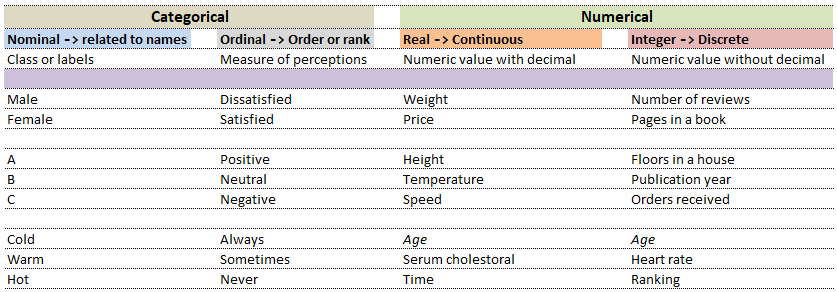• Big Data Analytics and Machine Learning
Divisions of Machine Learning
Topics have beeen categorized into logical sections that will guide beginners to moderately experienced users gain insights into machine learning methods.

Click on the links to access the content relevant to the topic!

⇓ ↡ ⇟ ⤋

Data Representation
Charts, Tables...

⇓ ↡ ⇟ ⤋

Basic Statistics
Mean, Median, Mode, Standard Deviation, Skewness, Normalization...

⇓ ↡ ⇟ ⤋

⇓ ↡ ⇟ ⤋

Linear Algebra and Hypothesis Testing
Statistics Test, Confidence Intervals, ANOVA, Type-1, Type-II Errors...

⇓ ↡ ⇟ ⤋

⇓ ↡ ⇟ ⤋

⇓ ↡ ⇟ ⤋

⇓ ↡ ⇟ ⤋

Reinforcement Learning
Agent, Environment, Reward, Penalty and Policy ... Q-Learning algorithm

⇓ ↡ ⇟ ⤋

Computer Vision
Image and Text Recognition...

⇓ ↡ ⇟ ⤋

Deep Learning
Convolution Neural Network...

##### Data

Computers understand data in a certain format whereas the nature of data can be numbers as well as words or phrases which cannot be quantified. For example, the difference in "positive and neutral" ratings cannot be quantified and will not be same as difference in "neutral and negative" ratings. There are many ways to describe the type of data we encounter in daily life such as (binary: either 0 or 1), ordered list (e.g. roll number or grade)...Note that even integers can be classified in the context they are used. This is demonstrated from following two examples.
 Nominal Ordinal What is your preferred mode of travel? How will you rate our services? 1 Flights 1 Satisfied 2 Trains 2 Neutral 3 Drive 3 Dissatisfied

While in the first case, digits 1, 2 and 3 are just variable labels [nominal scale] whereas in the second example, the same numbers (digits) indicate an order [ordinal scale].

Probability
What is the proabability or likelihood of a rainy day today? This is a very common question and weather forecasting companies use large number of historica and environment data to generate the probability of rain on a particular date. What if the forecast says 40% probability of rain on a particular day and it turns out to be a bright sunny day? Knowing the probability of an event does not mean we know will happen on any particular instance or occurrence. It only says that under certain conditions, 40% of the forecasts are correct in the long run. The Law of Large Numbers guarantees that this intuition is correct.

Law of Large Numbers

The relative frequeny of outcome of an event converges to a number, the probability of the outcome, as the number of observed outcomes increases. However, the LLN does not apply to every situation such as those with a pattern.

Conditional Probability

In Machine Learning, the general concept of 'probability' has less significance than the "conditional probability". A conditional probability is the chance of an event to occur once it is know that another even has occured. Bayes's rule shows how to get a conditional probability. This methd is used to classify e-mails as SPAM when certain keywords appear in the message or to estimate the probability that a viewer sees or skips the ads when he is watching a particular channel on YouTube.
Sample Scripts - Python, Excel VBA, MATLAB

Convert a text file into HTML code for a table

```import sys, os

file_name = str(sys.argv)
contents = open(file_name,"r")
i = 1
hdr = "S. No. Folder Name  \
File Name  Size  Unit  Pages  \n"
with open("textToTable.txt", "w") as oF:
for lines in contents.readlines():
td = lines.split()
oF.write("  %s "%str(i).zfill(5))
oF.write("  %s " %td)
oF.write("  %s " %td)
oF.write("  %s " %td)
oF.write("  %s " %td)
oF.write("  %s " %td)
oF.write("\n")
if (i%20 == 0):
oF.write(hdr)
i = i + 1```
List Contents of a Folder and Sub-folders
```# References:
# stackoverflow.com/questions/2104080/how-can-i-check-file-size-in-python
# www.geeksforgeeks.org/python-os-path-size-method
# www.geeksforgeeks.org/python-program-to-convert-a-list-to-string
# stackoverflow.com/questions/541390/extracting-extension-from-filename-in-python
# stackoverflow.com/questions/4226479/scan-for-secured-pdf-documents
# pythonexamples.org/python-if-not/
#------------------------------------------------------------------------------
import sys,os
from PyPDF2 import PdfFileReader

root = "F:\World_Hist_Books"
path = os.path.join(root, "targetdirectory")

'''
#------------------------------------------------------------------------------
#Get content of a directory: files, directories as LIST in the terminal
out_f = "List.txt"

#Write Only (‘w’) : Open the file for writing. If file already exits, data is
#truncated and over-written. The handle is positioned at the beginning of the
#file. Creates the file if it does not exist.
f = open(out_f, "w")     # f = open("List.txt", "w")

s = os.listdir()
for x in s:
#print(x)
f.write(x + '\n')
f.close
#------------------------------------------------------------------------------
'''
out_f = "fileList.txt"
f = open(out_f, "w")

def convert_bytes(num):  #bytes to kB, MB, GB
for x in ['bytes', 'KB', 'MB', 'GB', 'TB']:
if num < 1024.0:
return "%3.1f %s" % (num, x)
num = num / 1024.0

for path, subdirs, files in os.walk(root):
for name in files:
s = os.path.join(path, name)
b = os.path.getsize(os.path.join(path, name))
b = convert_bytes(b)

#Get number of pages in the PDF file: f.split(".")[-1]
ext = os.path.splitext(s)[1:].strip().lower()
nPg = 0

if (ext.upper() == "PDF"):
with open(s, 'rb') as pdf_file:
pdf_f = PdfFileReader(pdf_file)
if not pdf_f.isEncrypted:
nPg =  pdf_f.getNumPages()
pdf_file.close()

#Write complete path
#f.write(s)

#Replace \ with whitespace
A = s.split('\\')
f.write(' '.join(map(str, A)))
f.write(' ' + str(b) + '  ' + str(nPg) + '\n')

#Write only the file names
#f.write(s.split('\\')[-1] + '\n')

f.close

#------------------------------------------------------------------------------
# L is the list
#listToStr = ' '.join([str(elem) for elem in L])
#listToStr = ' '.join(map(str, L))
#print(listToStr)
#------------------------------------------------------------------------------```

The content on CFDyna.com is being constantly refined and improvised with on-the-job experience, testing, and training. Examples might be simplified to improve insight into the physics and basic understanding. Linked pages, articles, references, and examples are constantly reviewed to reduce errors, but we cannot warrant full correctness of all content.